Next: Example 10.5: The step-up Up: Inductance Previous: Example 10.3: The RL

## Example 10.4: The RC circuit

Question: A capacitor of capacitance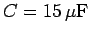is charged up to a voltage of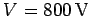, and then discharged by connecting a resistor of resistance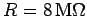across its terminals. How long does it take for the charge on the positive plate of the capacitor to be reduced to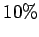of its original value?

Answer: Suppose that the resistor is first connected across the capacitor at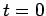. The chargeon the positive plate of the capacitor is given by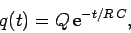which can be rearranged to give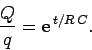Taking the natural logarithm of both sides, we obtain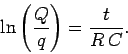Hence,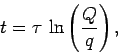where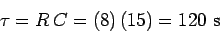is the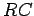time. Since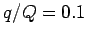, in this case, it follows that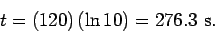Next: Example 10.5: The step-up Up: Inductance Previous: Example 10.3: The RL
Richard Fitzpatrick 2007-07-14